# CLASS 8 MATHS CHAPTER-3 UNDERSTANDING QUADRILATERALS

Exercise 3.1

Question 1.
Given here are some figures: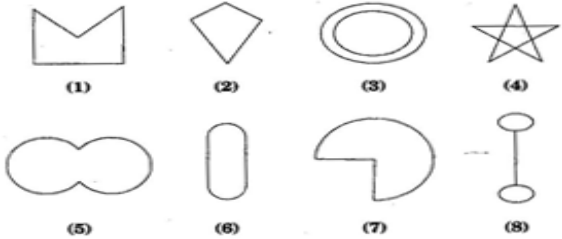Classify each of them on the basis of the following:
(a) Simple curve (b) Simple closed curve (c) Polygon (d) Convex polygon (e) Concave polygon

Solution :
(a) Simple curve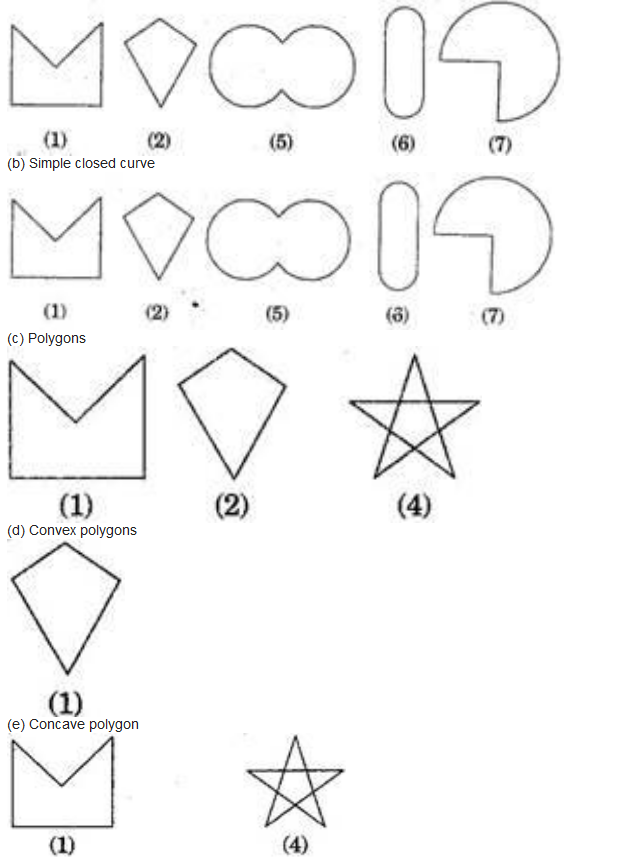(b) Simple closed curve

Question 2.
How many diagonals does each of the following have?
(b) A regular hexagon
(c) A triangle
Solution :
(a) A convex quadrilateral has two diagonals.
Here, AC and BD are two diagonals.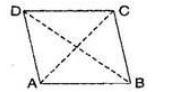(b) A regular hexagon has 9 diagonals.
Here, diagonals are AD, AE, BD, BE, FC, FB, AC, EC and FD.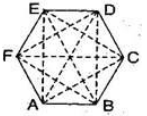(c) A triangle has no diagonal.
Question 3.
What is the sum of the measures of the angles of a convex quadrilateral? Will this property hold if the quadrilateral is not convex? (Make a non-convex quadrilateral and try)
Solution :
Let ABCD is a convex quadrilateral, then we draw a diagonal AC which divides the quadrilateral in two triangles.
∠A + ∠B + ∠C + ∠D
= ∠1 + ∠6 + ∠5 + ∠4 + ∠3 + ∠2
= (∠1 + ∠2 + ∠3) + (∠4 + ∠5 + ∠6)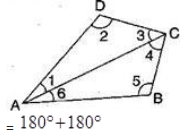[By Angle sum property of triangle]
360º
Hence, the sum of measures of the triangles of a convex quadrilateral is 360º
Yes, if quadrilateral is not convex then, this property will also be applied.
Let ABCD is a non-convex quadrilateral and join BD, which also divides the quadrilateral in two triangles.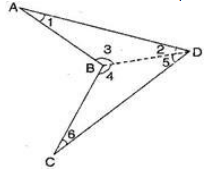Using angle sum property of triangle,
In △ABD, ∠1 + ∠2 + ∠3 = 180º;……….(i)
In △BDC, ∠4 + ∠5 + ∠6 = 180º;……….(i)
∠1 + ∠2 + ∠3 + ∠4 + ∠5 + ∠6 = 360º
⇒;∠1 + ∠2 + (∠3 + ∠4) + ∠5 + ∠6
= 360º
⇒;∠A + ∠B + ∠C + ∠D = 360º
Hence proved.
Question 4.
Examine the table. (Each figure is divided into triangles and the sum of the angles deduced from that.)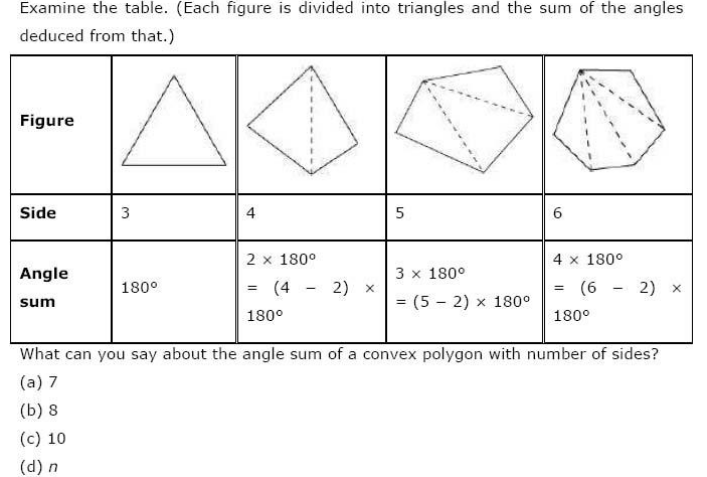Solution :
(a) When n = 7, then
Angle sum of a polygon =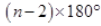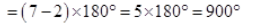(b) When n= 8, then
Angle sum of a polygon =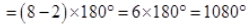(c) When n = 10, then
Angle sum of a polygon =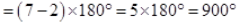(d) When n = n then
Angle sum of a polygon =Question 5.
What is a regular polygon? State the name of a regular polygon of:
(a) 3 sides
(b) 4 sides
(c) 6 sides
Solution :
A regular polygon: A polygon having all sides of equal length and the interior angles of equal size is known as regular polygon.
(i) 3 sides
Polygon having three sides is called a triangle.
(ii) 4 sides
Polygon having four sides is called a quadrilateral.
(iii) 6 sides
Polygon having six sides is called a hexagon.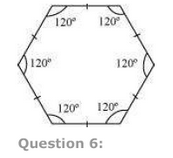x = 140º

(d)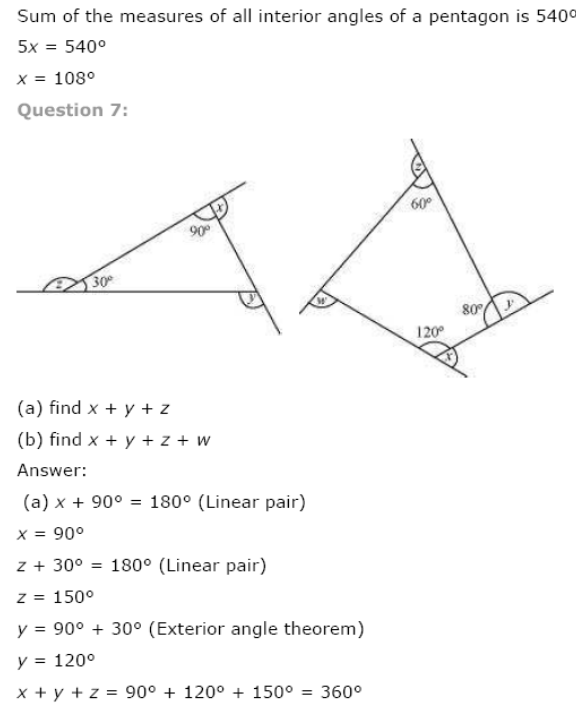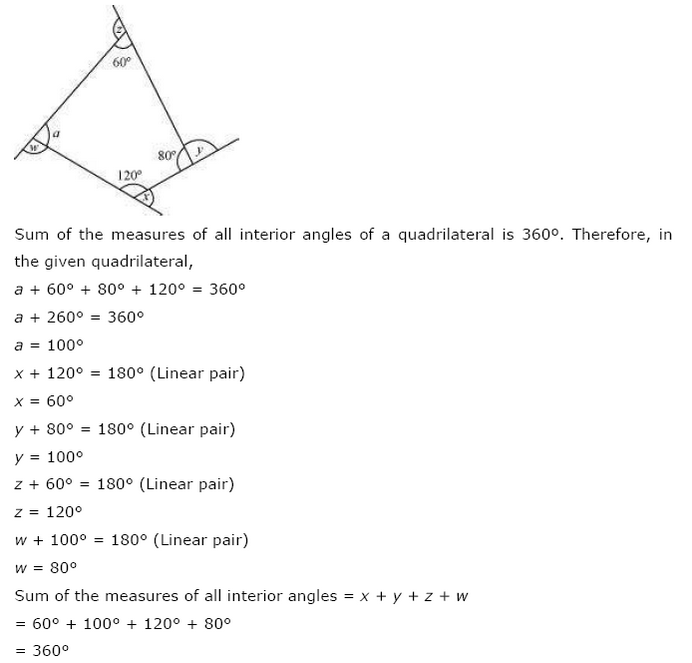NCERT Solutions for Class 8 Maths Exercise 3.2

Question 1.
Find x;in the following figures: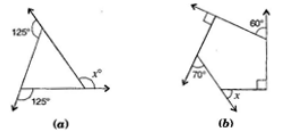Solution :
(a) Here,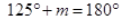[Linear pair]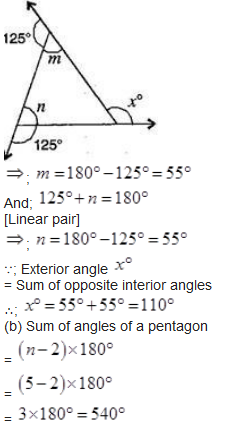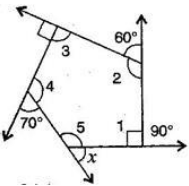By linear pairs of angles,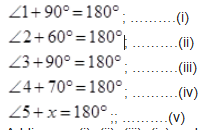Adding eq. (i), (ii), (iii), (iv) and (v),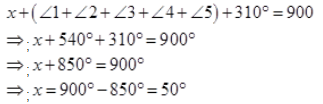Question 2.
Find the measure of each exterior angle of a regular polygon of:
(a) 9 sides
(b) 15 sides
Solution :
(i) Sum of angles of a regular polygon =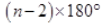=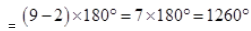Each interior angle =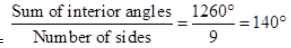Each exterior angle =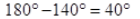(ii) Sum of exterior angles of a regular polygon = 360º
Each interior angle =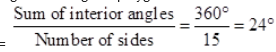Question 3.
How many sides does a regular polygon have, if the measure of an exterior angle is 24º?

Solution :
Let number of sides be  n.
Sum of exterior angles of a regular polygon = 360º
Number of sides =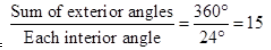Hence, the regular polygon has 15 sides.

Question 4.
How many sides does a regular polygon have if each of its interior angles is  165º?
Solution :
Let number of sides be n.
Exterior angle =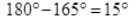Sum of exterior angles of a regular polygon = 360º
Number of sides =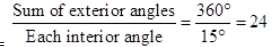Hence, the regular polygon has 24 sides.
Question 5.
(a) Is it possible to have a regular polygon with of each exterior angle as 22º?
(b) Can it be an interior angle of a regular polygon? Why?
Solution :
(a) No. (Since 22 is not a divisor of 360º)
(b) No, (Because each exterior angle is 180º –  22º = 158º; which is not a divisor of 360º)

Question 6.

(a) What is the minimum interior angle possible for a regular polygon? Why?
(b) What is the maximum exterior angle possible for a regular polygon?
Solution :
(a) The equilateral triangle being a regular polygon of 3 sides has the least measure of an
interior angle of 60º,
∵ Sum of all the angles of a triangle
180º
∴;   x + x +x = 180º
⇒     3 x = 180º
⇒       x  = 60º
(b) By (a), we can observe that the greatest exterior angle is   180º –  60º
= 120º.

NCERT Solutions for Class 8 Maths Exercise 3.3

Question 1.
Given a parallelogram ABCD. Complete each statement along with the definition or property used.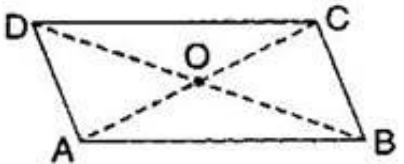(ii) ∠DCB = ______________
(iii) OC = _____________
(iv) m∠DAB + m∠CDA = ________

Solution :
[Since opposite sides of a parallelogram are equal]
(ii) ∠DCB = ∠DAB
[Since opposite angles of a parallelogram are equal]
(iii) OC = OA
[Since diagonals of a parallelogram bisect each other]
(iv) m∠DAB + m∠CDA = 180º
[Adjacent angles in a parallelogram are supplementary]

Question 2.
Consider the following parallelograms. Find the values of the unknowns x , y ,z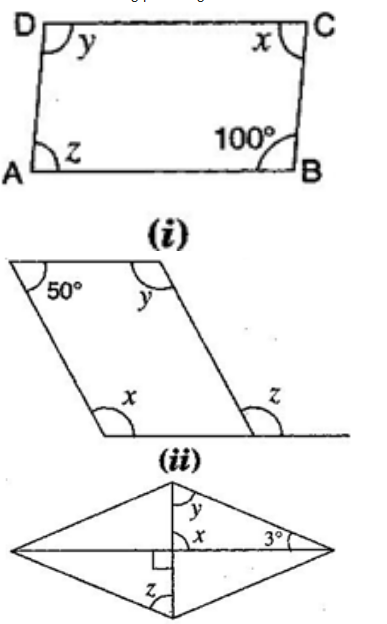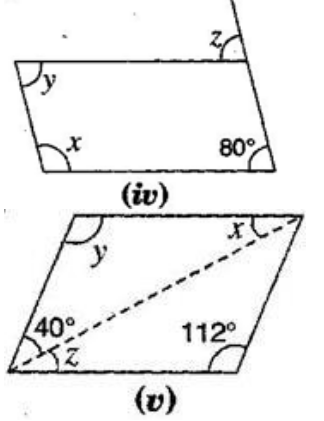Note: For getting correct answer, read  3º  =  30º in figure (iii)
Solution :
(i) ∠B + ∠C = 180º

[Adjacent angles in a parallelogram are supplementary]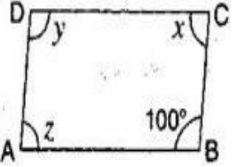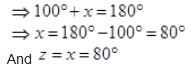[Since opposite angles of a parallelogram are equal]
Also   y = 100º
[Since opposite angles of a parallelogram are equal]
(ii)  x + 50º = 180º x = 50º = 180º
[Adjacent angles in a ||gm are supplementary]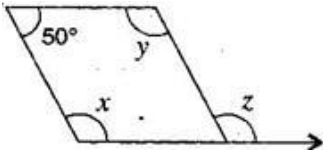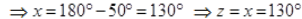[Corresponding angles]
(iii)  x = 90º
[Vertically opposite angles]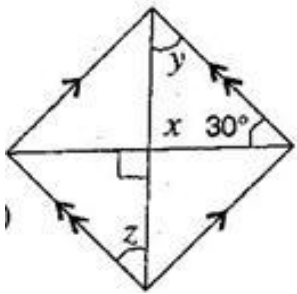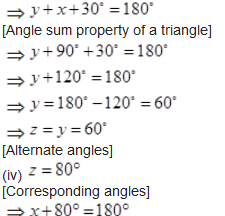[Adjacent angles in a ||gm are supplementary]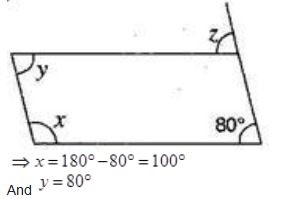[Opposite angles are equal in a ||gm]
(v)  y = 112º
[Opposite angles are equal in a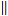gm]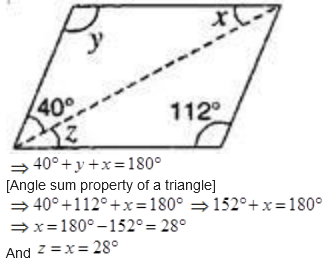[Alternate angles]

Question 3.

Can a quadrilateral ABCD be a parallelogram, if:
(i) ∠D + ∠B = 180º
(ii) AB = DC = 8 cm, AD = 4 cm and BC = 4.4 cm?
(iii) ∠A = 70º and ∠C = 65º?

Solution :
(i) ∠D + ∠B = 180º
It can be, but here, it needs not to be.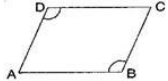(ii) No, in this case because one pair of opposite sides are equal and another pair of opposite sides are unequal. So, it is not a parallelogram.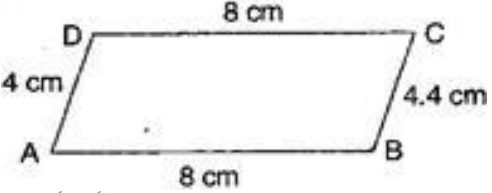(iii) No.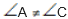Since opposite angles are equal in parallelogram and here opposite angles are not equal in quadrilateral ABCD. Therefore it is not a parallelogram.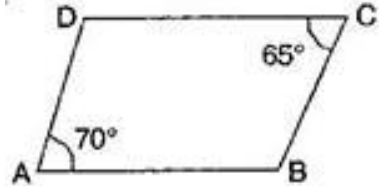Question 4.

Draw a rough figure of a quadrilateral that is not a parallelogram but has exactly two opposite angles of equal measures.

Solution :
ABCD is a quadrilateral in which angles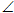A =C = 110º
Therefore, it could be a kite.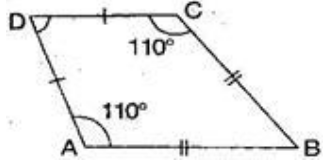Question 5.

The measure of two adjacent angles of a parallelogram are in the ratio 3 : 2. Find the measure of each of the angles of the parallelogram.

Solution :
Let two adjacent angles be 3X and 2X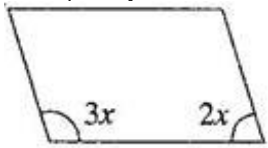Since the adjacent angles in a parallelogram are supplementary.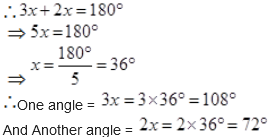Question 6.

Two adjacent angles of a parallelogram have equal measure. Find the measure of the angles of the parallelogram.

Solution : Let each adjacent angle be x,
Since the adjacent angles in a parallelogram are supplementary.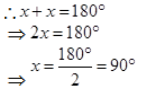Hence, each adjacent angle is 90º

Question 7.
The adjacent figure HOPW is a parallelogram. Find the angle measures  x , y and z State the properties you use to find them.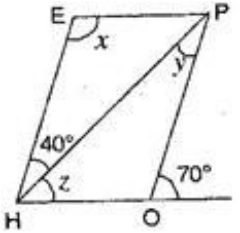∠HOP +  70º = 180º

Question 8.
The following figures GUNS and RUNS are parallelograms. Find x and (Lengths are in cm)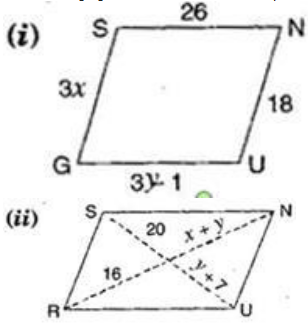Solution :

(i) In parallelogram GUNS,
GS = UN
[Opposite sides of parallelogram are equal]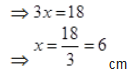Also GU = SN
[Opposite sides of parallelogram are equal]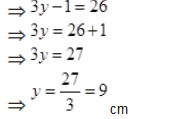Hence, x= 6 cm and x= 9 cm.
(ii) In parallelogram RUNS,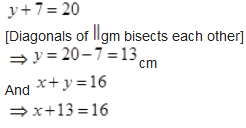⇒ x = 16 -13

⇒ x = 16 -13

⇒ x = 3cm

Hence,  x = 3 cm and y = 13cm.

Question 9.
In the figure, both RISK and CLUE are parallelograms. Find the value of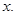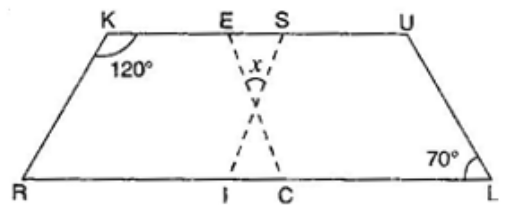Solution :

In parallelogram RISK,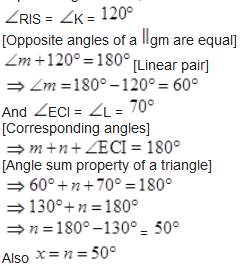[Vertically opposite angles]

Question 10.

Explain how this figure is a trapezium. Which is its two sides are parallel?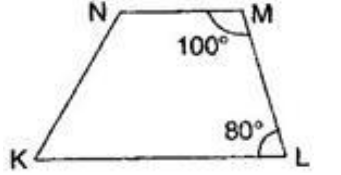Solution :
Here, ∠M + ∠L =  100º + 80º = 180º
[Sum of interior opposite angles is 180º]
NM and KL are parallel.
Hence, KLMN is a trapezium.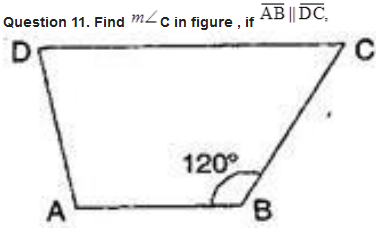Solution :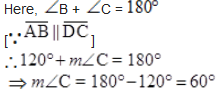Question 12.
Find the measure of ∠P and ∠S if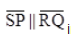in given figure.
(If you find m∠R is there more than one method to find m∠P)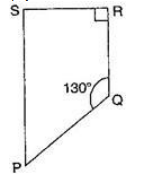Solution :

NCERT Solutions for Class 8 Maths Exercise 3.4

Get 30% off your first purchase!

X
error: Content is protected !!
Scroll to Top